A pocket handy formula sheet for maths, physics and chemistry all in the same app, with calculation for necessary formulas.

You can search any formulas

You can see the formulas without internet connection.
You can find all the essential formulas of Mathematics, Physics and Chemistry in one app.
You can find the details formulas of Math in another app All Math Formulas.
Periodic table included into Chemistry Formulas section
Suggestion and comments can be directed to sujitplus@gmail.com

Screenshots

•••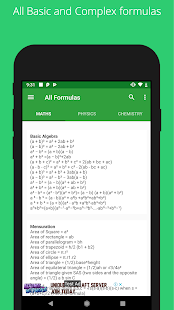•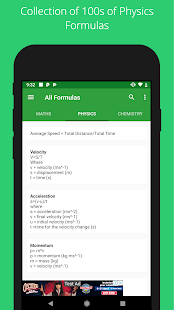•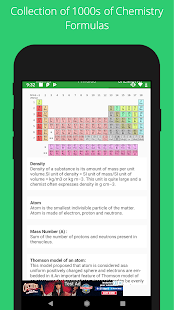•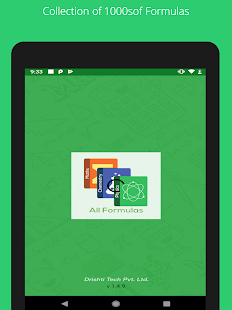••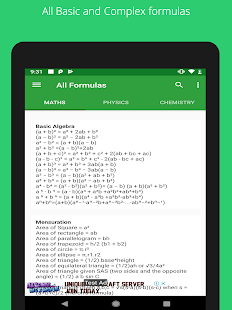••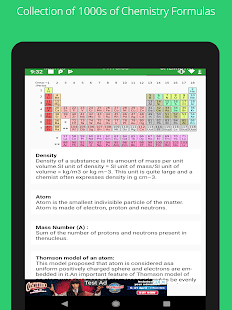••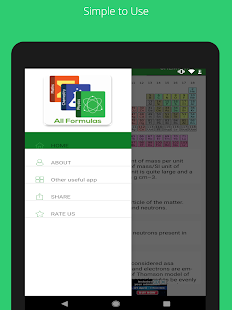•••